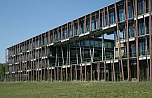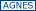# Humboldt Universitaet zu BerlinFaculty of Mathematics and Natural SciencesDepartment of Physicsauf deutsch

summer sem. 2022
Last update: 21.11.22 11:35:10

_SS 2023 WS 2022
SS 2022
SS 2021 WS 2021
Department of Physics
open chemistry

### 4020220122 Stochastic ThermodynamicsVL Mon 11-13 weekly NEW 15 2'101 (24) Mehrnaz Anvari, Jürgen Kurths

Digital- & Präsenz-basierter Kurs

classroom language
DE
requirements
Thermodynamics
structure / topics / contents
The course is divided to two parts. In the first part the concept of stochastic process, including continuous and discontinuous Markov processes, is
introduced. In the second part stochastic thermodynamics will be introduced, including the fundamental thermodynamics laws and the non-equilibrium thermodynamics concept in stochastic processes.

Course content
I. Stochastic Process (6 sessions)
§ Lecture 1: Introduction
- Brownian motion
§ Lecture 2: Continuous Markov Process
- Fokker-Planck equation
- Langevin equation
- Chapman-Kolmogorov equation
- Application: Turbulence, Earthquake
§ Lecture 3: Discontinuous Markov process I
- Differential Chapman-Kolmogorov (Master equation)
- Kramers-Moyal equation
- Pawula theorem
§ Lecture 4: Discontinuous Markov process II
- Jump-Diffusion equation
- Example: Epilepsy
II. Stochastic Thermodynamics (9 sessions)
§ Lecture 1: Equilibrium thermodynamics
- Review of equilibrium thermodynamics
§ Lecture 2: violation of second law of thermodynamics
- The perpetuum mobile of the second kind
- Maxwell’s Demon
- Feynman’s ratchet
- Szilard’s engine
§ Lecture 3: Thermodynamics laws for stochastic processes
- First law of thermodynamics for Fokker-Planck equation
- Second law of thermodynamics for Fokker-Planck equation
- Example: Colloidal particle
§ Lecture 4: Nonequilibrium thermodynamics I
- Detailed balance
- Fluctuation theorem, Detailed Fluctuation theorem, Integral
Fluctuation theorem